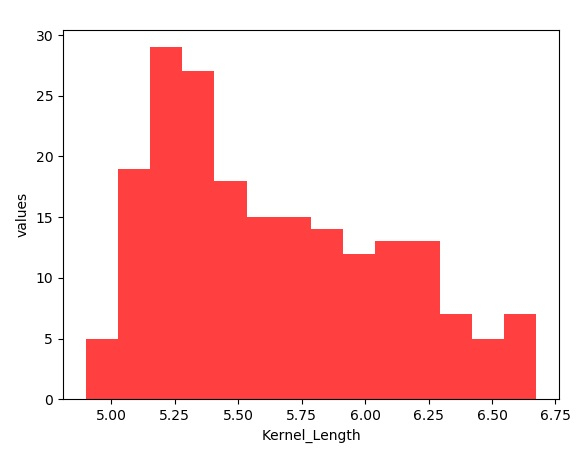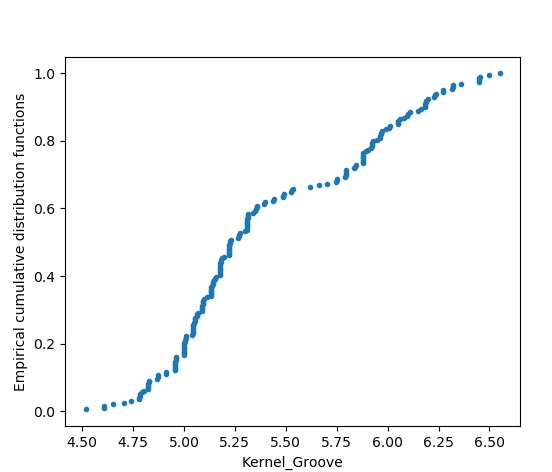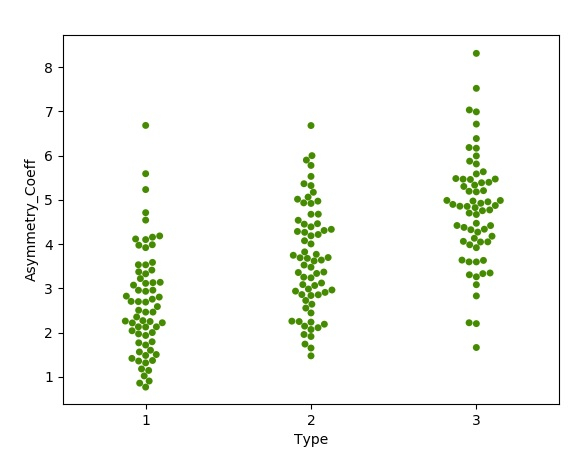# Statistical Thinking in Python

Statistics is fundamental to learn ml and AI. As Python is the language of choice for these Technologies, we will see how to write Python programs which incorporate statistical analysis. In this article we will see how to create graphs and charts using various Python modules. This variety of charts help us in analyzing the data quickly and deriving insides are conclusions graphically.

## Data Preparation

We take the data set containing the data about various seeds. This data set is available at kaggle in the link shown in the program below. It has eight columns which will be used to cerate various types of charts for comparing the features of different seeds. The below program loads the data set from the local environment and displays a sample of rows.

## Example

import pandas as pd
import warnings
warnings.filterwarnings("ignore")
#https://www.kaggle.com/jmcaro/wheat-seedsuci
print(datainput)

## Output

Running the above code gives us the following result −

      Area       Perimeter       Compactness    ...    Asymmetry.Coeff       Kernel.Groove       Type
0    15.26       14.84             0.8710       ...    2.221                      5.220             1
1    14.88       14.57             0.8811       ...    1.018                      4.956             1
2    14.29       14.09             0.9050       ...    2.699                      4.825             1
3    13.84       13.94             0.8955       ...    2.259                      4.805             1
4    16.14       14.99             0.9034       ...    1.355                      5.175             1
..     ...         ...             ...          ...    ...                         ...            ...
194   12.19      13.20             0.8783       ...    3.631                      4.870             3
195   11.23      12.88             0.8511       ...    4.325                      5.003             3
196   13.20      13.66             0.8883       ...    8.315                      5.056             3
197   11.84      13.21             0.8521       ...    3.598                      5.044             3
198   12.30      13.34             0.8684       ...    5.637                      5.063             3

[199 rows x 8 columns]

## Creating Histogram

To create a histogram we remove the header row from the csv file and read the file as a numpy array. Then we use the genfromtxt module to read the file. The kernel length filed is located as column index 3 in the array. Finally we use matplotlib to plot the histogram using the data set created by numpy and also apply the required labels.

## Example

import matplotlib.pyplot as plot
import numpy as np
from numpy import genfromtxt
seed_data = genfromtxt('E:\seeds.csv', delimiter=',')
Kernel_Length = seed_data[:, ]
x = len(Kernel_Length)
y = np.sqrt(x)
y = int(y)
z = plot.hist(Kernel_Length, bins=y, color='#FF4040')
z = plot.xlabel('Kernel_Length')
z = plot.ylabel('values')
plot.show()

## Output

Running the above code gives us the following result −## Empirical cumulative distribution functions

This chart shows the plot of the kernel groove size distributed across the data set. It is arranged from least to greatest value and it is shown as a distribution.

## Example

import matplotlib.pyplot as plot
import numpy as np
from numpy import genfromtxt
seed_data = genfromtxt('E:\seeds.csv', delimiter=',')
Kernel_groove = seed_data[:, 6]
def ECDF(seed_data):#Empirical cumulative distribution functions
i = len(seed_data)
m = np.sort(seed_data)
n = np.arange(1, i + 1) / i
return m, n
m, n = ECDF(Kernel_groove)
plot.plot(m, n, marker='.', linestyle='none')
plot.xlabel('Kernel_Groove')
plot.ylabel('Empirical cumulative distribution functions')
plot.show()

## Output

Running the above code gives us the following result −## Bee swarm plots

A beeswarm plot shows the size of a group of data points by visually clustering the each individual data point. We use the seaborn library to create this graph. We use the Type column from the data set to cluster similar type seeds together.

## Example

import pandas as pd
import matplotlib.pyplot as plot
import seaborn as sns
sns.swarmplot(x='Type', y='Asymmetry.Coeff',data=datainput, color='#458B00')#bee swarm plot
plot.xlabel('Type')
plot.ylabel('Asymmetry_Coeff')
plot.show()

## Output

Running the above code gives us the following result −Updated on: 04-Feb-2020

164 Views